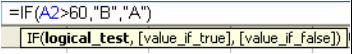top of page
Search

# IF Statements in Formulas in Microsoft Excel

A simple example of an IF statement is giving grades for percentages. We’ll assume that our percentage is in cell A2, and our formula resides in cell B2.An IF statement has at least 3 parts called arguments. When you type an IF statement, the tool tip automatically appears to tell you the 3 arguments required.The first part of the IF statement is called a logical test, which is also often referred to as a condition.

### Tip. Note that all "text" is surrounded by quotes when used in formulas. There are a few exceptions, such as True and False.

We like to think of the commas that separate the 3 arguments as words. The first comma means “give me”, and the second means “otherwise, give me”. So if we wrote the above formula in English instead, it would read like this:

If the value in cell A2 is greater than 60, give me a B, otherwise give me an A.If we don’t provide the third part of the argument, we simply get the “answer” returned to us: A2 is not greater than 60, so our condition or logical test is FALSE.

### Tip. You may only use 7 nested IF statements. That means 8 IFs can be in your formula; 7 being nested inside the first. Even in Excel 2003, there is no indication that the problem with the formula is too many IFs. If you have a requirement for more than 7, use VLOOKUP, instead.Let’s break down the formula, IF by IF:

• =IF(A1>60,”D”

If the value in A1 is greater than 60, put a D in cell B2.

• IF(A1>70,”C”

If the value in A1 is greater than 70, put a C in cell B2.

• IF(A1>80,”B”

If the value in A1 is greater than 80, put a B in cell B2.

• IF(A1>90,”A”

If the value in A1 is greater than 90, put an A in cell B2.

• “F”

Otherwise—if none of the above conditions are met—put an F in cell B2.

Combining IF with other formulas.

## IF with AND

Let’s suppose that a sales bonus is based on having made at least 20 sales call, and having made at least 15,000 in sales. We must check both conditions, using an AND statement.## IF with OR

Now, let’s use IF with an OR statement. In this case, a sales bonus is based on having made at least 20 sales calls, OR having made at least 15,000 in sales. If either condition is met, the bonus is given.## IF with OR and AND

Imagine that employees with perfect attendance become a member of an exclusive employee's club. When this occurs, they're given special consideration on bonuses. So, in this case, if they're a member OR they sell more than 15,000 and make at least 20 sales calls, they receive the bonus.Source: Microsoft

The Tech Platform